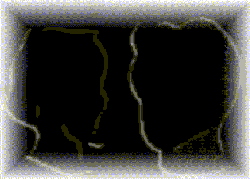8Created  Within Radford University's Gender Studies Website == 1996 == == 1997 == == 1998 == == 1999 == == 2000 == == 2001 == == 2002 == == 2005 == == 2006 == == 2007 ==   == 2008 ==   == 2009 == ==   2005-Change in Format    ====      == == 2009  == == 2010  == == 2011  == == 2012  == == 2014  == == 2016  == == 2016f == == 2017  == == 2018g == == 2018K == == 2019   == Invited Speakers   Sample of Student Conference Participants   Gender Studies  Psychology     College of Humanities & Behavioral Sciences     Radford University         Web Page by: W. Andrew   Last updated  September 19 th  2022 Copyright © 2006 W. Andrew